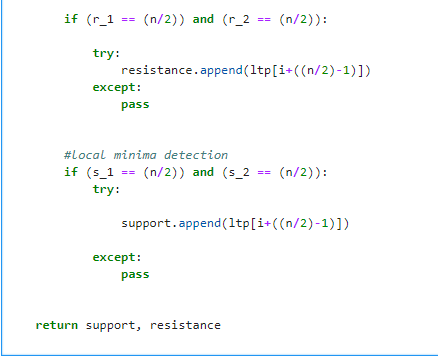# A Simple Python Function to Detect Support/Resistance Levels

edited September 2017
I wrote this simple function to detect support/resistance levels. The algorithm is very simple actually. All it does is smoothens the curve and detects local minima and maxima levels. Any suggestions to improve this?
``def supres(ltp, n):    """    This function takes a numpy array of last traded price    and returns a list of support and resistance levels     respectively. n is the number of entries to be scanned.    """    from scipy.signal import savgol_filter as smooth    #converting n to a nearest even number    if n%2 != 0:        n += 1        n_ltp = ltp.shape    # smoothening the curve    ltp_s = smooth(ltp, (n+1), 3)     #taking a simple derivative    ltp_d = np.zeros(n_ltp)    ltp_d[1:] = np.subtract(ltp_s[1:], ltp_s[:-1])     resistance = []    support = []        for i in xrange(n_ltp - n):        arr_sl = ltp_d[i:(i+n)]        first = arr_sl[:(n/2)] #first half        last = arr_sl[(n/2):] #second half                r_1 = np.sum(first > 0)        r_2 = np.sum(last < 0)        s_1 = np.sum(first < 0)        s_2 = np.sum(last > 0)        #local maxima detection        if (r_1 == (n/2)) and (r_2 == (n/2)):             resistance.append(ltp[i+((n/2)-1)])        #local minima detection        if (s_1 == (n/2)) and (s_2 == (n/2)):             support.append(ltp[i+((n/2)-1)])    return support, resistance``
Tagged:
kudos if you designed it ..get approach to determine S&R
• edited May 2018
Thanks, do you have any example?
• edited May 2018
nice code...
• how we can use this ????
• Hi, thanks for the code. what exactly is n that needs to pass here. Can you pls explain that a bit.
• n is the number of entries to be scanned
• Nice approach, I prefer going the historical touch and reverse route. i.e. calculating based on previous closes that made the price go up or down. The more touch and reverse or close below and fall points there are for certain prices the more confidence in the S/R.
• edited December 2019
Hi:

A minor amendment in the function.

In the case there is no support or resistance, the function doesn't run properly.

To fix it, we need to add try/pass before the end of the function. The return will be an empty list, which is fine.

Follows the amendment:Thanks,

Arthur
• Ok I looked at the code and what he is trying to do is

1. From points, he derived smooth curve
2. Calculated delta for slope = dy/dx and as time between two candles is constant, dx = 1
3. ltp_d is dy/dx wth dx = 1, so dy (ltp_d, means ltp delta) is nothing but difference of adjacent prices
4. Then a window of n candles arr_sl is panned over ltp_d array
5. And during this panning, at any point, where the mid point of this window has positive and negative delta count same, means it is minima or maxima
6. If first half count is positive and second half is negative, it means maxima and visa versa

Use this 1 minute candle ltp to find and then trade on 5 minute candle chart

Or use this for 30 minute candle to then use result to trade on positional

Catch is that you must use this for candles of time much lower than your trading candle chart

• Hi! Great algorithm. I tried this and the other one here https://stackoverflow.com/questions/8587047/support-resistance-algorithm-technical-analysis

What would be a good value for 'n' when working with daily close prices here for positional trades?

Thanks!